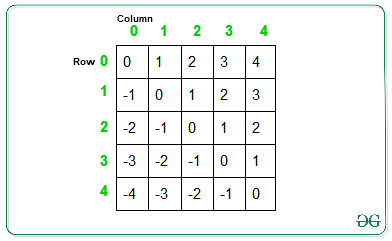# Sort a 2D vector diagonally

Given a 2D vector of NxM integers. The task is to sort the elements of the vectors diagonally from top-left to bottom-right in decreasing order.

Examples:

Input: arr[][] = { { 10, 2, 3 }, { 4, 5, 6 }, {7, 8, 9 } }
Output:
10 2 0
4 9 0
0 0 5

Input: arr[][] = { { 10, 2, 43 }, { 40, 5, 16 }, { 71, 8, 29 }, {1, 100, 5} }
Output:
29 2 0
8 10 0
71 5 5
0 0 0

## Recommended: Please try your approach on {IDE} first, before moving on to the solution.

Approach:
Observations:The above images shows the difference between the column index and row index at each cell. The cells having same difference from top-left to bottom-down cell forms a diagonal.
Below are the steps to sort diagonal in decreasing order:

1. Store the diagonal element with positive difference in one Array of Vectors(say Pos[]) such that elements at the cell having difference(say a) is stored at index a of Pos[] array.
2. Store the diagonal element with negative difference in another Array of Vectors(say Neg[]) such that elements at the cell having difference(say -b) is stored at index abs(-b) = b of Neg[] array.
3. Sort both the Array of Vectors increasing order.
4. Traverse the given 2D vector and updated the value at current cell with value stored in Pos[] and Neg[] array.
• If difference between column and row index(say d) is positive, then updated the value from Pos[d] array and remove the last elment as:
```d = i - j
arr[i][j] = Pos[d][Pos.size()-1]
Pos[d].pop_back()
```
• If difference between column and row index(say d) is negative, then updated the value from Neg[d] array and remove the last element as:
```d = j - i
arr[i][j] = Neg[d][Neg.size()-1]
Neg[d].pop_back()
```

Below is the implementation of the above approach:

 `// C++ program to sort the 2D vector ` `// diagonally in decreasing order ` `#include "bits/stdc++.h" ` `using` `namespace` `std; ` ` `  `// Function that sort the elements ` `// of 2D vector ` `void` `diagonalSort(vector >& mat) ` `{ ` ` `  `    ``// Calculate the rows and column ` `    ``int` `row = mat.size(); ` `    ``int` `col = mat.size(); ` ` `  `    ``// Array of vectors to store the ` `    ``// diagonal elements ` `    ``vector<``int``> Neg[row]; ` `    ``vector<``int``> Pos[col]; ` ` `  `    ``// Traverse the 2D vector and put ` `    ``// element in Array of vectors at ` `    ``// index difference between indexes ` `    ``for` `(``int` `i = 0; i < row; i++) { ` `        ``for` `(``int` `j = 0; j < col; j++) { ` ` `  `            ``// If diff is negative, then ` `            ``// push element to Neg[] ` `            ``if` `(j < i) { ` `                ``Neg[i - j].push_back(mat[i][j]); ` `            ``} ` ` `  `            ``// If diff is positive, then ` `            ``// push element to Pos[] ` `            ``else` `if` `(j > i) { ` `                ``Pos[j - i].push_back(mat[i][j]); ` `            ``} ` ` `  `            ``// If diff is 0, then push ` `            ``// element to Pos ` `            ``else` `{ ` `                ``Pos.push_back(mat[i][j]); ` `            ``} ` `        ``} ` `    ``} ` ` `  `    ``// Sort the Array of vectors ` `    ``for` `(``int` `i = 0; i < row; i++) { ` `        ``sort(Neg[i].begin(), Neg[i].end()); ` `    ``} ` `    ``for` `(``int` `i = 0; i < col; i++) { ` `        ``sort(Pos[i].begin(), Pos[i].end()); ` `    ``} ` ` `  `    ``// Update the value to arr[][] ` `    ``// from the sorted Array of vectors ` `    ``for` `(``int` `i = 0; i < row; i++) { ` `        ``for` `(``int` `j = 0; j < col; j++) { ` ` `  `            ``// If diff is positive ` `            ``if` `(j < i) { ` `                ``int` `d = i - j; ` `                ``int` `l = Neg[d].size() - 1; ` `                ``mat[i][j] = Neg[d][l - 1]; ` `                ``Neg[d].pop_back(); ` `            ``} ` ` `  `            ``// If diff is negative ` `            ``else` `if` `(j > i) { ` `                ``int` `d = j - i; ` `                ``int` `l = Pos[d].size() - 1; ` `                ``mat[i][j] = Pos[d][l - 1]; ` `                ``Pos[d].pop_back(); ` `            ``} ` ` `  `            ``// If diff is 0 ` `            ``else` `{ ` `                ``int` `l = Pos.size(); ` `                ``mat[i][j] = Pos[l - 1]; ` `                ``Pos.pop_back(); ` `            ``} ` `        ``} ` `    ``} ` `} ` ` `  `// Function to print element ` `void` `printElement(vector >& arr) ` `{ ` ` `  `    ``// Traverse the 2D vector ` `    ``for` `(``int` `i = 0; i < arr.size(); i++) { ` `        ``for` `(``int` `j = 0; j < arr.size(); j++) { ` `            ``cout << arr[i][j] << ``' '``; ` `        ``} ` `        ``cout << endl; ` `    ``} ` `} ` ` `  `// Driver Code ` `int` `main() ` `{ ` `    ``vector > arr = { { 10, 2, 3 }, ` `                                 ``{ 4, 5, 6 }, ` `                                 ``{ 7, 8, 9 } }; ` `    ``diagonalSort(arr); ` ` `  `    ``// Function call to print elements ` `    ``printElement(arr); ` `} `

Output:

```10 2 0
4 9 0
0 0 5
```

Time Complexity: O(N2)

GeeksforGeeks has prepared a complete interview preparation course with premium videos, theory, practice problems, TA support and many more features. Please refer Placement 100 for details

My Personal Notes arrow_drop_upCheck out this Author's contributed articles.

If you like GeeksforGeeks and would like to contribute, you can also write an article using contribute.geeksforgeeks.org or mail your article to contribute@geeksforgeeks.org. See your article appearing on the GeeksforGeeks main page and help other Geeks.

Please Improve this article if you find anything incorrect by clicking on the "Improve Article" button below.

Article Tags :
Practice Tags :

2

Please write to us at contribute@geeksforgeeks.org to report any issue with the above content.